# An Approach to the Triangle

#### Top Of Page

 Section I Introduction & Key Findings Section II Triangles in general Section III The Area of the Triangle { A-O-T } Section IV The { Congruent-Triangles Tetrahedron } Section V Shape Classifications based on the { Fermat Point } Section VI An Infinite Series of { Triangles } Section VII The { Nine Circles } of the Triangle Section VIII Mapping { Triangles } onto { Triangles } Section IX The { Sine-Square } & { Cosine-Square } Functions Section X Concluding Remarks Appendix A Brief Notes on the { Fermat Point } Appendix B { Centroid } for { Triangle D-E-F } & { Triangle R-S-T }

### Section I --- Introduction & Key Findings

In { An Approach to the Three Body Problem } back in 1996 , it was not determined as to how the 3 Degrees-Of-Freedom ( DOF's ) associated with a triangle should be broken-down into :

• 1 { Degree-Of-Freedom } associated with the size , &
• 2 { Degrees-Of Freedom } associated with the shape .

This paper is an effort towards a better understanding of the { Triangle } .

#### ONE. --- The { C-T Tetrahedron }

• The { C-T Tetrahedron } , or { Congruent-Triangles Tetrahedron } , is a tetrahedron whose four (4) outer-surfaces are { mutually-congruent triangles } .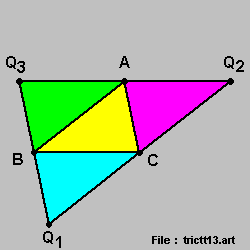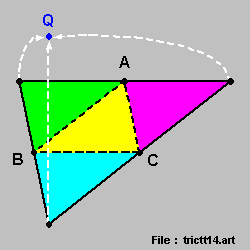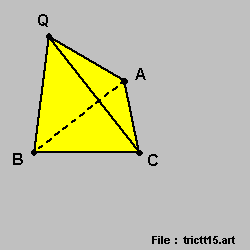We start-off with any triangle , { Triangle Q1-Q2-Q3 } , and :

• we identify the mid-points as { Point A } , { Point B } , & { Point C } , respectively ,

• we then join the mid-points to produce 4 { congruent triangles } , as shown in the diagram on-the-left ,

• we then fold the 3 corners upwards , creasing along the black dotted-lines as marked in the diagram in-the-middle ,

• to meet at a single point , { Point Q } ,

• and we end up with the { C-T Tetrahedron } as shown in the diagram on-the-right .

( note : the { Right-Angle Triangle } is a special case . )

• The { C-T Tetrahedron } then exhibits true { Quad-Symmetry } :

• if we were to toss the { C-T Tetrahedron } and let it land on a table-top / horizontal-surface ,

then , no matter which of the 4 surfaces it lands on :

• the { shape & size } of the contact-surface will always be the same ,

• the { shape & size } of the { landed-object } will always { look-the-same } .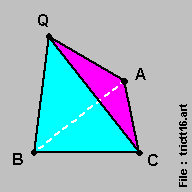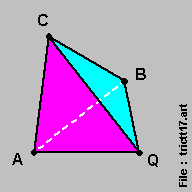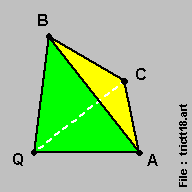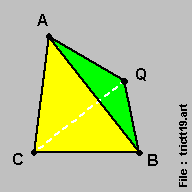• The { C-T Tetrahedron } also has a very special property :

• one single & unique point , within the { C-T Tetrahedron } , serves as :

• the { center-point } for the { circumscribing sphere } passing thru the 4 nodal-points ,
• the { center-point } for the { inscribing sphere } just-touching the 4 surfaces , &
• the { centroid } for the { C-T Tetrahedron } ,
• the { Fermat Point } , where the { sum of the distances to the 4 nodal-points } is { at-a-minimum } .

Rather interesting !

#### TWO. --- An Infinite Series of { Triangles }

• We construct an infinite series of { Triangles } :

• starting-off with a triangle of any size & shape , and

• we repeated apply the traditional { Fermat Point Construction Method } of attaching 3 { equilateral triangles } to the 3 Sides , so that :

• the outer-vertices of the 3 { equilateral triangles } form the 3 vertices of a new triangle , and this is the next member of the infinite series .

The infinite series converges onto the { equilateral triangle } .

• This then 'opens-the-door' for other infinite series of Triangles / other Geometric Shapes .

#### THREE. --- Matching { Fermat Point } onto { Fermat Point }

• In the { Three Body Problem } , the { Center of Mass } is always important because its position can be readily predicted ,

• arising from the { conservation of linear momentum } .

• We then construct , for the 3 { mass's } :

• the physical { Position-Vectors Triangle } and the { Mass * Position-Vector Triangle } ,

with the { centroid } of the latter being the { Center of Mass } .

• Our proposal here is to do the mapping between the 2 triangles via [ matching { Fermat Point } on { Fermat Point } ] , so that :

• the 3 { 120-degrees-apart Fermat-Point-Directions } are super-imposed on one-another for the 2 triangles .

• Our { Shape Classification System } here is { Fermat Point } based ,

• and this offered a great convenience as { 3-colinear-points triangles } are always included .

#### FOUR. --- Triangles with Integer Sides

• For { Triangles with Integer Sides } , the values for { Sine-Square } & { Cosine-Square } are always { whole-fractions } for all 3 angles .

This then poses 2 open questions :

• We can now close-in on the size of an angle via successive comparisons with { Triangles with Interger Sides } .

Is this a better , or complimentary , definition to { defining an angle via the arc-length } ?

• A triangle splits [ 180 degrees ] into 3 complimentary parts .

Does { Triangles with Integer Sides } hold any special insights on numbers , prime & non-prime alike ?